# Linear Inequations

## Exercise 4

#### Question 1

Solve the inequation 3x - 11 ≤ 3, where x ∈ {1, 2, 3, .........., 10}. Also represent its solution on number line.

Given,

$3x-11 \leq 3 \\[0.5em] \Rightarrow 3x-11 + 11 \leq 3 + 11 \\[0.5em] \Rightarrow 3x \leq 14 \\[0.5em] \Rightarrow x \leq \dfrac{14}{3} \\[0.5em] \Rightarrow x \leq 4.66$

But x ∈ {1, 2, 3, ..........,, 10}

∴ Solution Set = {1, 2, 3, 4}.

The graph of the solution set is shown by thick dots on the number line.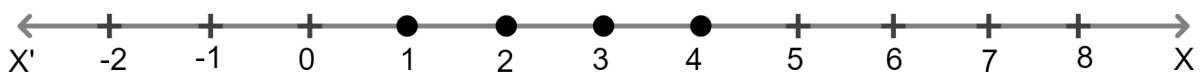#### Question 2

Solve 2(x - 3) < 1, x ∈ {1, 2, 3, .........., 10}.

Given,

$2(x - 3) \lt 1 \\[0.5em] \Rightarrow 2x-6 \lt 1 \\[0.5em] \Rightarrow 2x-6 + 6 \lt 1 + 6 \\[0.5em] \Rightarrow 2x \lt 7 \\[0.5em] \Rightarrow x \lt \dfrac{7}{2} \\[0.5em] \Rightarrow x \lt 3.5$

But x ∈ {1, 2, 3, ……., 10}
Solution set is {1, 2, 3}.

#### Question 3

Solve 5 - 4x > 2 - 3x, x ∈ W . Also represent its solution on number line.

Given,

$5 - 4x \gt 2 - 3x \\[0.5em] \Rightarrow 5 - 2 \gt -3x + 4x \\[0.5em] \Rightarrow 3 \gt x \\[0.5em] \Rightarrow x \lt 3$

Hence, Solution set is {0, 1, 2}.

The graph of the solution set is shown by thick dots on the number line.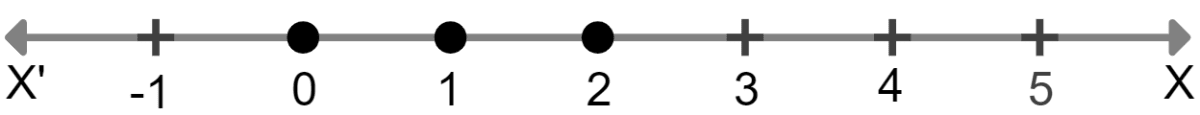#### Question 4

List the solution set of 30 – 4(2x – 1) < 30, given that x is a positive integer.

Given,

$30 - 4(2x - 1) \lt 30 \\[0.5em] \Rightarrow 30-8x+4 \lt 30 \\[0.5em] \Rightarrow 34-8x \lt 30 \\[0.5em] \Rightarrow 34-30 \lt 8x \\[0.5em] \Rightarrow 8x \gt 4 \\[0.5em] \Rightarrow x \gt \dfrac{1}{2}$

Since , x is a positive integer
x = {1, 2, 3, 4, …..}.

#### Question 5

Solve : 2(x – 2) < 3x – 2, x ∈ { –3, –2, –1, 0, 1, 2, 3} .

Given,

$2(x – 2) \lt 3x – 2 \\[0.5em] \Rightarrow 2x – 4 \lt 3x – 2 \\[0.5em] \Rightarrow 2x – 3x \lt – 2 + 4 \\[0.5em] \Rightarrow – x \lt 2 \\[0.5em] \Rightarrow x \gt – 2 \\[0.5em]$

Solution set = {–1, 0, 1, 2, 3} .

#### Question 6

If x is a negative integer, find the solution set of $\dfrac{2}{3}+\dfrac{1}{3} (x + 1) \gt 0$.

Given,

$\dfrac{2}{3}+\dfrac{1}{3} (x + 1) \gt 0 \\[0.5em] \Rightarrow \dfrac{2}{3} +\dfrac{x}{3} + \dfrac{1}{3} \gt 0 \\[0.5em] \Rightarrow \dfrac{x}{3} + 1 \gt 0 \\[0.5em] \Rightarrow \dfrac{x}{3} \gt – 1 \\[0.5em] \Rightarrow x \gt -3$

x is a negative integer Solution set = {-2, –1}.

#### Question 7

Solve x – 3(2 + x) > 2(3x - 1), x ∈ {-3, -2, -1, 0, 1, 2}. Also represent its solution on the number line.

Given,

$x - 3(2 + x) \gt 2(3x - 1) \\[0.5em] \Rightarrow x - 6 - 3x \gt 6x - 2 \\[0.5em] \Rightarrow x - 3x - 6x \gt - 2 + 6 \\[0.5em] \Rightarrow - 8x \gt 4 \\[0.5em] \Rightarrow 8x \lt -4 \\[0.5em] \Rightarrow x \lt -\dfrac{1}{2}$

Since, x ∈ { -3, -2, -1, 0, 1, 2}
Hence, Solution set = { -3, -2, -1}.

The graph of the solution set is shown by thick dots on the number line.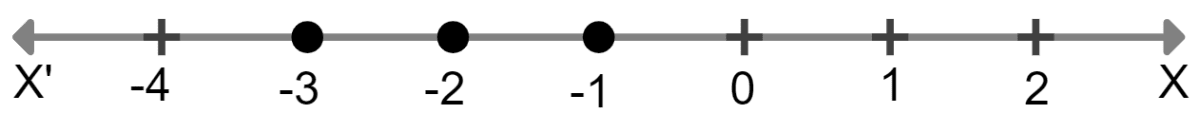#### Question 8

Given x ∈ {1, 2, 3, 4, 5, 6, 7, 9} solve x – 3 < 2x – 1.

Given,

$x - 3 \lt 2x - 1\\[0.5em] \Rightarrow x - 2x \lt - 1 + 3\\[0.5em] \Rightarrow - x \lt 2\\[0.5em] \Rightarrow x \gt - 2$

Since, x ∈ {1, 2, 3, 4, 5, 6, 7, 9}

Solution set = {1, 2, 3, 4, 5, 6, 7, 9}.

#### Question 9

List the solution set of the inequation

$\dfrac{1}{2} + 8x \gt 5x -\dfrac{3}{2}, x ∈ \bold{Z}$

Given, $\dfrac{1}{2} +8x \gt 5x - \dfrac{3}{2} \\[0.5em] \Rightarrow 8x-5x \gt - \dfrac{3}{2}-\dfrac{1}{2} \\[0.5em] \Rightarrow 3x \gt - \dfrac{4}{2} \\[0.5em] \Rightarrow 3x \gt -2 \\[0.5em] \Rightarrow x \gt -\dfrac{2}{3}$

Since , x ∈ Z
Solution set = {0, 1, 2, 3, 4…..}

#### Question 10

List the solution set of:

$\dfrac{11-2x}{5} \ge \dfrac{9-3x}{8} + \dfrac{3}{4}, x ∈ \bold{N}.$

Given,

$\dfrac{11-2x}{5} \ge \dfrac{9-3x}{8} + \dfrac{3}{4} \\[0.5em] \Rightarrow \dfrac{11-2x}{5} \ge \dfrac{9 - 3x + 6}{8} \\[0.5em] \Rightarrow 8(11 - 2x) \ge 5(15 - 3x) \\[0.5em] \Rightarrow 88 - 16x \ge 75 - 15x \\[0.5em] \Rightarrow - 16x + 15x \ge 75 - 88 \\[0.5em] \Rightarrow - x \ge - 13 \\[0.5em] \Rightarrow x ≤ 13 \\[0.5em]$

Since, x ∈ N
Solution set = {1, 2, 3, 4, 5, ..... , 13}.

#### Question 11

Find the values of x, which satisfy the inequation:

$−2 \le \dfrac{1}{2}-\dfrac{2x}{3} \le 1\dfrac{5}{6}$, x ∈ N.

Graph the solution set on the number line.

Given,

$-2 \le \dfrac{1}{2} - \dfrac{2x}{3} \le 1\dfrac{5}{6} \\[0.5em] \Rightarrow -2 \le \dfrac{1}{2}-\dfrac{2x}{3} \le \dfrac{11}{6} \\[0.5em] \Rightarrow -2-\dfrac{1}{2} \le \dfrac{1}{2} - \dfrac{2x}{3} - \dfrac{1}{2} \le \dfrac{11}{6} - \dfrac{1}{2}\\[0.5em] \text {By subtracting } \dfrac{1}{2} \text{ on both sides of inequality in the above line.}\\[0.5em] \Rightarrow -\dfrac{5}{2} \le -\dfrac{2x}{3} \le \dfrac{8}{6} \\[0.5em] \Rightarrow -\dfrac{5}{2} \times 6 \le -\dfrac{2x}{3}\times 6 \le \dfrac{8}{6}\times 6 \text{ (Multiplying complete equation with 6) } \\[0.5em] \Rightarrow -15 \le -4x \le 8 \\[0.5em] \Rightarrow 15 \ge 4x \ge -8 \\[0.5em] \Rightarrow \dfrac{15}{4} \ge x \ge -2$

Since x ∈ N,

∴ Solution Set = {1, 2, 3}

The graph of the solution set is shown by thick dots on the number line.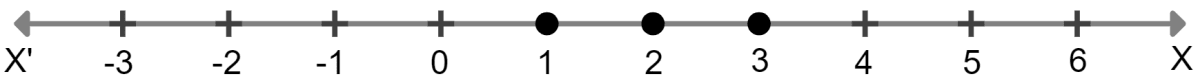#### Question 12

If x ∈ W, find the solution set of $\dfrac{3}{5} x - \dfrac{2x−1}{3} \gt$ 1. Also graph the solution set on the number line, if possible.

Given,

$\dfrac{3}{5}x-\dfrac{2x−1}{3} \gt 1\\[0.5em] \Rightarrow \dfrac{9x - 10x + 5}{15} \gt 1 \text{ [Taking LCM as 15]} \\[0.5em] -x + 5 \gt 15 \\[0.5em] \Rightarrow – x \gt 15 – 5\\[0.5em] \Rightarrow – x \gt 10\\[0.5em] \Rightarrow x \lt – 10\\[0.5em]$

But x ∈ W
Solution set = Φ
Hence it can't be represented on number line.

#### Question 13(i)

Solve:

$\dfrac{x}{2} + 5 \le \dfrac{x}{3} + 6$ where x is a positive odd integer.

Given,

$\dfrac{x}{2} +5 \le \dfrac{x}{3} + 6\\[0.5em] \Rightarrow \dfrac{x}{2} - \dfrac{x}{3} \le 6-5\\[0.5em] \Rightarrow \dfrac{3x-2x}{6} \le 1\\[0.5em] \Rightarrow \dfrac{x}{6} \le 1\\[0.5em] \Rightarrow x \le 6$

Since, x is a positive odd integer
Hence, x = {1, 3, 5}.

#### Question 13(ii)

Solve:

$\dfrac{2x+3}{3} \ge \dfrac{3x−1}{4}$ where x is positive even integer.

Given,

$\dfrac{2x+3}{3} \ge \dfrac{3x−1}{4}\\[0.5em] \Rightarrow \dfrac{2x}{3} + \dfrac{3}{3} \ge \dfrac{3x}{4}-\dfrac{1}{4}\\[0.5em] \Rightarrow \dfrac{2x}{3} + 1 \ge \dfrac{3x}{4} - \dfrac{1}{4}\\[0.5em] \Rightarrow \dfrac{2x}{3} - \dfrac{3x}{4} \ge -\dfrac{1}{4}-1\\[0.5em] \Rightarrow \dfrac{8x-9x}{12} \ge - \dfrac{5}{4}\\[0.5em] \Rightarrow -\dfrac{x}{12} \ge -\dfrac{5}{4}\\[0.5em] \Rightarrow \dfrac{x}{12} \le \dfrac{5}{4}\\[0.5em] \Rightarrow x \le \dfrac{5}{4} \times 12\\[0.5em] \Rightarrow x \le 15$

Since, x is a positive even integer.
x = {2, 4, 6, 8, 10, 12, 14}.

#### Question 14

Given that x ∈ I, solve the inequation and graph the solution on the number line :

$3 \ge \dfrac{x−4}{2} + \dfrac{x}{3} \ge 2$

Given,

$3 \ge \dfrac{x−4}{2}+ \dfrac{x}{3} \ge 2\\[2em] \text{ Solving left side: }\\[1em] 3 \ge \dfrac{x−4}{2}+ \dfrac{x}{3} \\[0.5em] \Rightarrow 3 \ge + \dfrac{3x-12+2x}{6}\\[0.5em] \Rightarrow 3 \ge + \dfrac{5x-12}{6}\\[0.5em] \Rightarrow 18 \ge 5x-12\\[0.5em] \Rightarrow 5x-12 \le 18\\[0.5em] \Rightarrow 5x \le 30\\[0.5em] \Rightarrow x \le 6\\[1em] \text{Solving right side:} \\[1em] \dfrac{x−4}{2} + \dfrac{x}{3} ≥ 2\\[0.5em] \Rightarrow \dfrac{3x-12+2x}{6} \ge 2 \\[0.5em] \Rightarrow \dfrac{5x-12}{6} \ge 2\\[0.5em] \Rightarrow 5x-12 \ge 12 \\[0.5em] \Rightarrow 5x \ge 24\\[0.5em] \Rightarrow x \ge \dfrac{24}{5}\\[0.5em] \Rightarrow x \ge 4\dfrac{4}{5} \\[0.5em]$

∴ Solution Set = {5, 6}.

The graph of the solution set is shown by thick dots on the number line.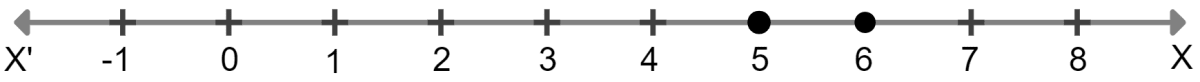#### Question 15

Solve 1 ≥ 15 - 7x > 2x - 27, x ∈ N.

Given,

$1 \ge 15 - 7x \gt 2x - 27\\[0.5em] \Rightarrow 1 \ge 15 - 7x \text{ and } 15 - 7x \gt 2x - 27\\[0.5em] \Rightarrow 7x \ge 15-1 \text{ and } -7x-2x \gt -27-15\\[0.5em] \Rightarrow 7x \ge 14 \text{ and } -9x \gt -42\\[0.5em] \Rightarrow x \ge 2 \text{ and } -x \gt -\dfrac{42}{9}\\[0.5em] \Rightarrow x \ge 2 \text{ and } x \lt \dfrac{14}{3}\\[0.5em] \Rightarrow 2 \le x \lt \dfrac{14}{3}$

Since x ∈ N,

Solution set = {2, 3, 4}.

#### Question 16

If x ∈ Z, solve 2 + 4x < 2x – 5 ≤ 3x. Also represent its solution on the number line.

Given,

$2 + 4x \lt 2x - 5 \le 3x\\[0.5em] \Rightarrow 2 + 4x \lt 2x - 5 \text{ and } 2x - 5 \le 3x \\[0.5em] \Rightarrow 4x - 2x \lt - 5 - 2 \text{ and } 2x - 3x \le 5\\[0.5em] \Rightarrow 2x \lt -7 \text{ and } -x \le 5\\[0.5em] \Rightarrow x \lt -\dfrac{7}{2} \text{ and } x \ge -5\\[0.5em] \Rightarrow -5\le x \lt -\dfrac{7}{2}$

Since x ∈ Z,

∴ Solution set = {-5, -4}.

The graph of the solution set is shown by thick dots on the number line.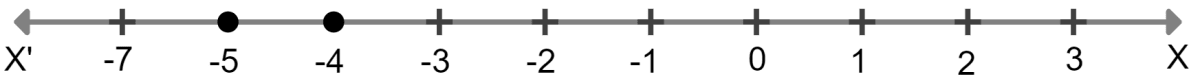#### Question 17

Solve : $\dfrac{4x-10}{3} \le \dfrac{5x-7}{2}, x ∈ \bold{R}$ and represent the solution set on the number line.

Given,

$\dfrac{4x−10}{3} \le \dfrac{5x−7}{2}\\[0.5em] \Rightarrow \dfrac{4x-10}{3} \times 6 \le \dfrac{5x-7}{2} \times 6 \text{ ( Multiplying both sides by 6) }\\[0.5em] \Rightarrow 8x - 20 \le 15x - 21$

$\Rightarrow 8x-15x \le -21 +20\\[0.5em] \Rightarrow -7x \le -1\\[0.5em] \Rightarrow 7x \ge 1\\[0.5em] \Rightarrow x \ge \dfrac{1}{7}$

∴ Solution Set = {x : x ∈ R, x ≥ $\dfrac{1}{7}$}

The graph of the solution set is represented by thick black line starting from and including $\dfrac{1}{7}$ on the number line.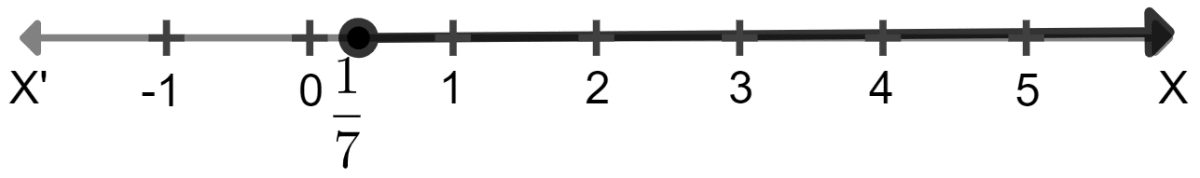#### Question 18

Solve $\dfrac{3x}{5} - \dfrac{2x-1}{3} \gt 1, x ∈ \bold{R}$ and represent the solution set on the number line.

Given,

$\dfrac{3x}{5} - \dfrac{2x-1}{3} \gt 1 \\[0.5em] \Rightarrow 9x - (10x - 5) \gt 15 \\[0.5em] \Rightarrow 9x - 10x + 5 \gt 15 \\[0.5em] \Rightarrow - x \gt 15 - 5 \\[0.5em] \Rightarrow - x \gt 10 \\[0.5em] \Rightarrow x \lt -10$

x ∈ R.
Hence , Solution set = {x : x ∈ R, x < –10}.

The graph of the solution set is represented by thick black line starting from -10 (not including -10) on the number line.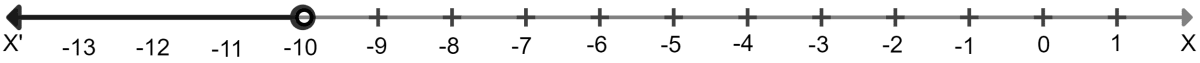#### Question 19

Given that x ∈ R, solve the following inequation and graph the solution on the number line:

-1 ≤ 3 + 4x < 23

Given,

$-1 \le 3 + 4x \lt 23 \\[0.5em] \Rightarrow – 1 – 3 \le 4x \lt 23 – 3 \\[0.5em] \Rightarrow – 4 \le 4x \lt 20 \\[0.5em] \Rightarrow – 1 \le x \lt 5, x ∈ \bold{R} \\[0.5em]$

Solution Set = {x : x ∈ R, -1 ≤ x < 5}

The graph of the solution set is represented by thick black line starting from -1 ( including -1) till 5 ( not including 5 ) on the number line.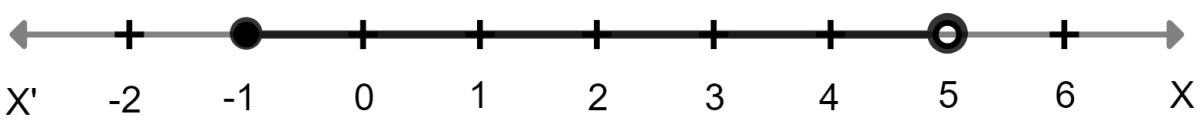#### Question 20

Solve the following inequation and graph the solution on the number line.

$-2\dfrac{2}{3} \le x + \dfrac{1}{3} \lt 3 + \dfrac{1}{3}$, x ∈ R.

Given, $-2 \dfrac{2}{3} \le x + \dfrac{1}{3} \lt 3+ \dfrac{1}{3} \\[0.5em] \Rightarrow -\dfrac{8}{3} \le x + \dfrac{1}{3} \lt \dfrac{10}{3}$

On multiplying the equation by 3 , we get,

$\Rightarrow -8 \le 3x + 1 \lt 10\\[0.5em] \Rightarrow -8-1 \le 3x + 1 -1 \lt 10 - 1 \text{ (adding -1 to equation)} \\[0.5em] \Rightarrow -9 \le 3x \lt 9 \\[0.5em] \Rightarrow -3 \le x \lt 3 \\[0.5em]$

∴ Solution set = {x : x ∈ R , -3 ≤ x < 3}.

The graph of the solution set is represented by thick black line starting from -3 ( including -3) till 3 ( not including 3 ) on the number line.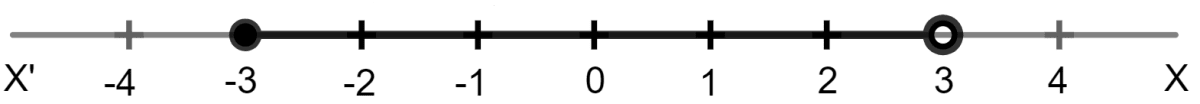#### Question 21

Solve the following inequation and represent the solution set on the number line :

$-3 \lt - \dfrac{1}{2} -\dfrac{2x}{3} \le \dfrac{5}{6}, x ∈ \bold{R}.$

First solving the left equation

$-3 \lt - \dfrac{1}{2} −\dfrac{2x}{3} \\[0.5em] \Rightarrow -3 \lt -(\dfrac{1}{2} + \dfrac{2x}{3}) \\[0.5em] \Rightarrow -\Big(\dfrac{1}{2} + \dfrac{2x}{3}\Big) \gt -3 \\[0.5em] \Rightarrow −\dfrac{2x}{3} \gt -3 + \dfrac{1}{2} \\[0.5em] \Rightarrow −\dfrac{2x}{3} \gt \dfrac{-5}{2} \\[0.5em] \Rightarrow \dfrac{2x}{3} \lt \dfrac{5}{2} \\[0.5em] \Rightarrow x \lt \dfrac{15}{4} \text{ .......(1)}$

Now the right side equation

$-\dfrac{1}{2} - \dfrac{2x}{3} \le \dfrac{5}{6}\\[0.5em] \Rightarrow - \dfrac{2x}{3} \le \dfrac{5}{6} + \dfrac{1}{2} \\[0.5em] \Rightarrow - \dfrac{2x}{3} \le \dfrac{5+3}{6}\\[0.5em] \Rightarrow - \dfrac{2x}{3} \le \dfrac{8}{6} \\[0.5em] \Rightarrow \dfrac{2x}{3} \ge -\dfrac{8}{6}\\[0.5em] \Rightarrow x \ge \dfrac{-8 \times 3}{6 \times 2}\\[0.5em] \Rightarrow x \ge -2 \text{ .......(2)}$

From 1 and 2 we get,

$-2 \le x \lt \dfrac{15}{4}$
∴ Solution set ={x : x ∈ R , -2 $\le x \lt \dfrac{15}{4}$}

The graph of the solution set is represented by thick black line starting from -2 (including -2) till $\dfrac{15}{4}$ (excluding $\dfrac{15}{4}$) on the number line.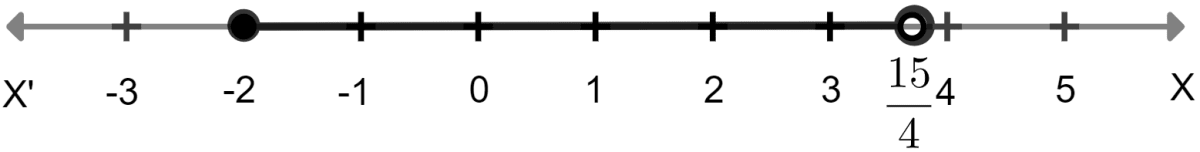#### Question 22

Solving the following inequation, write the solution set and represent it on the number line.

$–3(x - 7) \ge 15 - 7x \gt \dfrac{x+1}{3}, x ∈ \bold{R}.$

Given,

$–3(x - 7) \ge 15 - 7x \gt \dfrac{x+1}{3}, x ∈ \bold{R}$

Solving left side

$-3(x-7) \ge 15 - 7x \\[0.5em] \Rightarrow -3x + 21 \ge 15 - 7x \\[0.5em] \Rightarrow -3x + 7x \ge 15 - 21 \\[0.5em] \Rightarrow 4x \ge -6 \\[0.5em] \Rightarrow x \ge -\dfrac{6}{4} \\[0.5em] \Rightarrow x \ge -\dfrac{3}{2}$

Solving right side

$15 -7x \gt \dfrac{x+1}{3} \\[0.5em] \Rightarrow 15- \dfrac{1}{3} \gt \dfrac{x}{3} + 7x \\[0.5em] \Rightarrow \dfrac{44}{3} \gt \dfrac{22x}{3} \\[0.5em] \Rightarrow \dfrac{44 \times 3}{22 \times 3} \gt x \\[0.5em] \Rightarrow x \lt 2$

∴ Solution Set = {x : x ∈ R, $-\dfrac{3}{2}$ ≤ x < 2}

The graph of the solution set is represented by thick black line starting from and including $-\dfrac{3}{2}$ till 2 (not including 2) on the number line.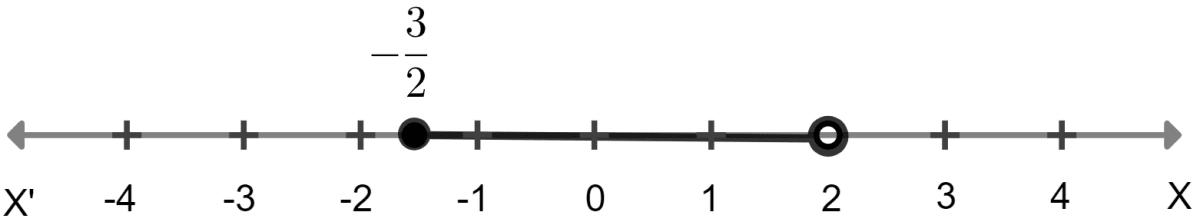#### Question 23

Solve the following inequation, write down the solution set and represent it on the real number line:

-2 + 10x ≤ 13x + 10 < 24 + 10x, x ∈ Z.

Given,

$−2 + 10x \le 13x + 10 \lt 24 + 10x \\[1em] \text{ Solving left side } \\[0.5em] -2 + 10x \le 13x + 10 \\[0.5em] \Rightarrow 10x -13x \le 10 +2\\[0.5em] \Rightarrow -3x \le 12 \\[0.5em] \Rightarrow 3x \ge -12 \\[0.5em] \Rightarrow x \ge -4 \\[1em] \text { Solving right side } \\[0.5em] 13x + 10 \lt 24 + 10x \\[0.5em] \Rightarrow 13x -10x \lt 24 -10 \\[0.5em] \Rightarrow 3x \lt 14 \\[0.5em] \Rightarrow x \lt \dfrac{14}{3}$

∴ Solution Set = {x : x ∈ Z , -4 ≤ x < $\dfrac{14}{3}$} = {-4, -3, -2, -1, 0, 1, 2, 3, 4}

The graph of the solution set is represented by thick black dots.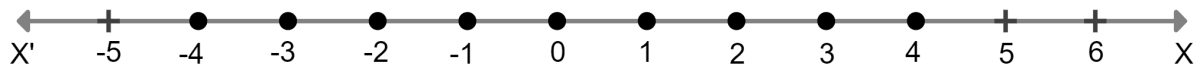#### Question 24

Solve the inequation 2x – 5 ≤ 5x + 4 < 11, where x ∈ I. Also represent the solution set on the number line.

Given,

$2x - 5 \le 5x + 4 \lt 11 \\[0.5em] \Rightarrow 2x - 5 \le 5x + 4 \text { and } 5x + 4 \lt 11 \\[0.5em] \Rightarrow 2x - 5 - 4 \le 5x \text { and } 5x + 4 \lt 11 \\[0.5em] \Rightarrow 2x - 9 \le 5x \text{ and } 5x \lt 11 - 4 \\[0.5em] \Rightarrow 2x - 5x \le 9 \text{ and } 5x \lt 7 \\[0.5em] \Rightarrow -3x \le 9 \text{ and } x \lt \dfrac{7}{5} \\[0.5em] \Rightarrow 3x \ge - 9 \text{ and } x \lt \dfrac{7}{5} \\[0.5em] \Rightarrow x \ge - 3 \text{ and } x \lt 1.4 \\[0.5em] \therefore -3 \le x \lt 1.4$

∴ Solution set = {-3, -2, -1, 0, 1}

The graph of the solution set is represented by thick black dots on the number line.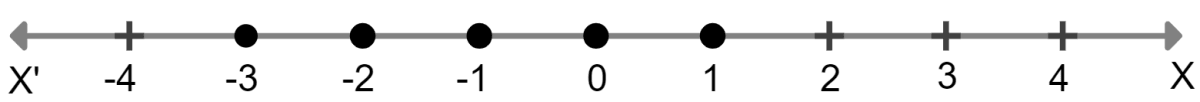#### Question 25

If x ∈ I, A is the solution set of 2(x - 1) < 3x - 1 and B is the solution set of 4x – 3 ≤ 8 + x, find A ∩ B.

Given,

$2 (x - 1) \lt 3 x - 1 \\[0.5em] \Rightarrow 2x - 2 \lt 3x - 1 \\[0.5em] \Rightarrow 2x - 3x \lt - 1 + 2 \\[0.5em] \Rightarrow - x \lt 1 \\[0.5em] \Rightarrow x \gt - 1 \\[0.5em] \therefore \text{A} = \lbrace 0, 1, 2, 3, ....\rbrace$

Also,

$4x - 3 \le 8 + x \\[0.5em] \Rightarrow 4x - x \le 8 + 3 \\[0.5em] \Rightarrow 3x \le 11 \\[0.5em] \Rightarrow x \le \dfrac{11}{3} \\[0.5em] \therefore \text{B} = \lbrace....., -1, 0, 1, 2, 3\rbrace$

∴ A ∩ B = {0, 1, 2, 3}

#### Question 26

If P is the solution set of -3x + 4 < 2x - 3, x ∈ N and Q is the solution set of 4x - 5 < 12, x ∈ W, find

(i) P ∩ Q

(ii) Q – P

First finding solution set P

$-3 x + 4 \lt 2 x - 3 \\[0.5em] \Rightarrow - 3x - 2x \lt -3-4 \\[0.5em] \Rightarrow - 5x \lt - 7 \\[0.5em] \Rightarrow 5x \gt 7 \\[0.5em] \Rightarrow x \gt \dfrac{7}{5}$

∴ P = {2, 3, 4, 5, ..... }

Finding solution set Q

$\Rightarrow 4x-5 \lt 12 \\[0.5em] \Rightarrow 4x \lt 12 + 5 \\[0.5em] \Rightarrow 4x \lt 17 \\[0.5em] \Rightarrow x \lt \dfrac{17}{4}$

∴ Q = {4, 3, 2, 1, 0}

(i) P ∩ Q = {2, 3, 4}

(ii) Q – P = {0, 1}

#### Question 27

A = {x : 11x - 5 > 7x + 3, x ∈ R } and
B = {x : 18x - 9 ≥ 15 + 12x, x ∈ R }.
Find the range of set A ∩ B and represent it on a number line.

$\text{A} = {x : 11x - 5 \gt 7x + 3, x ∈ \bold{R}} \\[0.5em] \text{B} = {x : 18x - 9 \ge 15 + 12x, x ∈ \bold{R}} \\[1em] \text{Now, A} = 11x - 5 \gt 7x + 3 \\[0.5em] \Rightarrow 11x - 7x \gt 3 + 5 \\[0.5em] \Rightarrow 4x \gt 8 \\[0.5em] \Rightarrow x \gt 2, x ∈ \bold{R} \\[1em] B= 18x - 9 \ge 15 + 12x \\[0.5em] \Rightarrow 18x -12x \ge 15 + 9 \\[0.5em] \Rightarrow 6x \ge 24 \\[0.5em] \Rightarrow x \ge 4 \\[0.5em] \therefore \text{A} ∩ \text{B} = {x : x ∈ \bold{R}, x \ge 4}$

The graph is represented by a thick back line starting from 4 (including 4)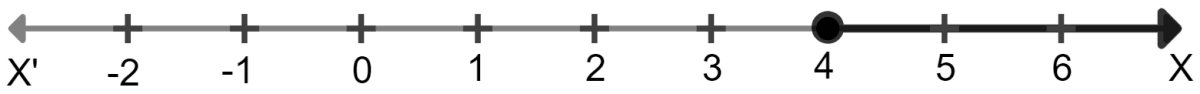#### Question 28

Given: P = {x : 5 < 2x - 1 ≤ 11, x ∈ R}
Q = {x : - 1 ≤ 3 + 4x < 23, x ∈ I} where R = {real numbers}, I = {integers}.
Represent P and Q on number line. Write down the elements of P ∩ Q.

Given,

P = {x : 5 < 2x - 1 ≤ 11, x ∈ R}

$\Rightarrow 5 \lt 2x - 1 \le 11$

Solving left side,

$5 \lt 2x -1 \\[0.5em] \Rightarrow 6 \lt 2x \\[0.5em] \Rightarrow 3 \lt x \\[0.5em] \Rightarrow x \gt 3 \\[0.5em]$

Solving right side,

$2x -1 \le 11 \\[0.5em] \Rightarrow 2x \le 12 \\[0.5em] \Rightarrow x \le 6$

∴ P = {x : x ∈ R, 3 < x ≤ 6}.

The graph of the solution set of P is represented by thick black line starting from 3 (not including 3) till 6 (including 6).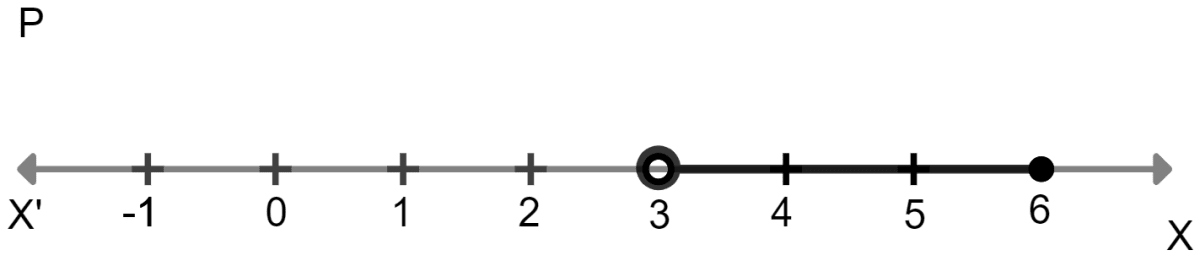Given,

Q = {x : - 1 ≤ 3 + 4x < 23, x ∈ I}

$\Rightarrow -1 \le 3 + 4x \lt 23 \\[0.5em] \Rightarrow -1 \le 3 + 4x \text{ and } 3 + 4x \lt 23 \\[0.5em] \Rightarrow -4x \le 3 + 1 \text{ and } 4x \lt 20 \\[0.5em] \Rightarrow -4x \le 4 \text{ and } x \lt 5 \\[0.5em] \Rightarrow -x \le 1 \text{ and } x \lt 5 \\[0.5em] \Rightarrow x \ge -1 \text{ and } x \lt 5$

∴ Q = {x : x ∈ I, -1 ≤ x < 5} = {-1, 0, 1, 2, 3, 4}.

The graph of the solution set of Q is represented by thick black dots.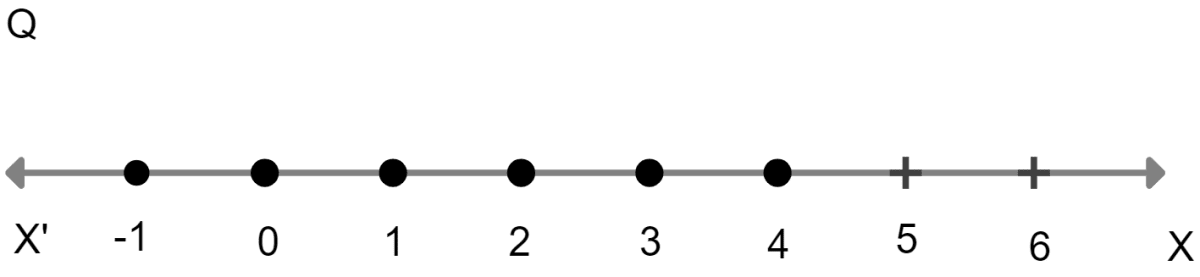∴ P ∩ Q = {4}

#### Question 29

If x ∈ I, find the smallest value of x which satisfies the inequation

$2x +\dfrac{5}{2} \gt \dfrac{5x}{3} + 2$

Given,

$2x + \dfrac{5}{2} \gt \dfrac{5x}{3} + 2 \\[0.5em] \Rightarrow 2x - \dfrac{5x}{3} \gt 2 - \dfrac{5}{2} \\[0.5em] \Rightarrow 12x - 10x \gt 12 - 15 \text{ (Multiplying both sides by 6)} \\[0.5em] \Rightarrow 2x \gt - 3 \\[0.5em] \Rightarrow x \gt - \dfrac{3}{2}$

∴ Smallest value of x which satisfies this inequation is -1

#### Question 30

Given 20 – 5x < 5(x + 8), find the smallest value of x, when

(i) x ∈ I

(ii) x ∈ W

(iii) x ∈ N

Given,

$20 - 5 x \lt 5 (x + 8) \\[0.5em] \Rightarrow 20 - 5x \lt 5x + 40 \\[0.5em] \Rightarrow - 5x - 5x \lt 40 - 20 \\[0.5em] \Rightarrow - 10x \lt 20 \\[0.5em] \Rightarrow - x \lt 2 \\[0.5em] \Rightarrow x \gt - 2 \\[0.5em]$

(i) When x ∈ I, then smallest value = -1.

(ii) When x ∈ W, then smallest value = 0.

(iii) When x ∈ N, then smallest value = 1.

#### Question 31

Solve the following inequation and represent the solution set on the number line:

$4x - 19 \lt \dfrac{3x}{5} - 2 \le - \dfrac{2}{5} + x, x ∈ \bold{R}$

Given,

$4x-19 \lt \dfrac{3x}{5} - 2 \le - \dfrac{2}{5} + x, x ∈ \bold{R} \\[1em] 4x - 19 \lt \dfrac{3x}{5} -2 \text{ and } \dfrac{3x}{5} - 2 \le - \dfrac{2}{5} + x \\[0.5em] \Rightarrow 4x -\dfrac{3x}{5} \lt -2 + 19 \text{ and } \dfrac{3x}{5} - 2 \le - \dfrac{2}{5} + x \\[0.5em] \Rightarrow \dfrac{20x-3x}{5} \lt 17 \text{ and } -2 + \dfrac{2}{5} \le x - \dfrac{3x}{5} \\[0.5em] \Rightarrow \dfrac{17x}{5} \lt 17 \text{ and } \dfrac{-8}{5} \le \dfrac{2x}{5} \\[0.5em] \Rightarrow \dfrac{x}{5} \lt 1 \text{ and } x \ge -4 \\[0.5em] \Rightarrow x \lt 5 \text{ and } x \ge -4 \\[0.5em] \Rightarrow -4 \le x \lt 5 , x ∈ \bold{R}$

Hence, Solution set = {x : x ∈ R, -4 ≤ x < 5}.

The graph of the solution set is represented by thick line starting from -4 (including -4) till 5 (not including 5).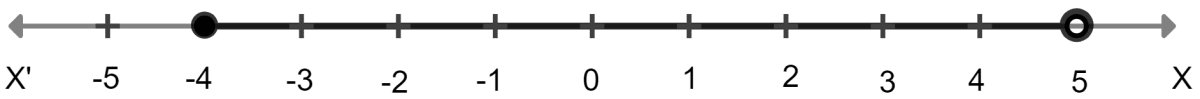#### Question 32

Solve the given inequation and graph the solution on the number line :

2y - 3 < y + 1 ≤ 4y + 7; y ∈ R

Given,

$2y - 3 \lt y + 1 \le 4y + 7; y ∈ \bold{R}. \\[0.5em] \text{ (a) Solving left side } \\[0.5em] 2y - 3 \lt y + 1 \\[0.5em] \Rightarrow 2y - y \lt 1 + 3 \\[0.5em] \Rightarrow y \lt 4 \\[0.5em] \Rightarrow 4 \gt y \\[0.5em] \text{(b) Solving right side } \\[0.5em] y + 1 \le 4y + 7 \\[0.5em] \Rightarrow y - 4y \le 7 - 1 \\[0.5em] \Rightarrow -3y \le 6 \\[0.5em] \Rightarrow 3y \ge -6 \\[0.5em] \Rightarrow y \ge -2$

∴ Solution Set = {y : y ∈ R, -2 ≤ y < 4}

The graph of the solution set is represented by a thick black line starting from -2 (including -2) till 4 (not including 4).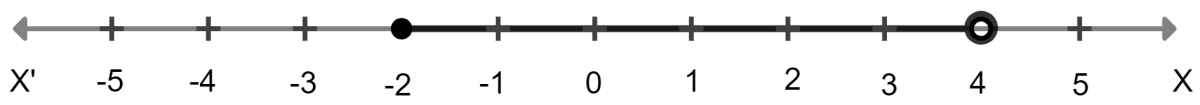#### Question 33

Solve the inequation and represent the solution set on the number line.

$-3 + x \le \dfrac{8x}{3} + 2 \le \dfrac{14}{3} + 2x, \text{where } x ∈ \bold{I}.$

Solving left side:

$-3 + x \le \dfrac{8x}{3} + 2 \\[0.5em] \Rightarrow -3 -2 \le \dfrac{8x}{3} -x \\[0.5em] \Rightarrow -3 -2 \le \dfrac{8x-3x}{3} \\[0.5em] \Rightarrow -5 \le \dfrac{5x}{3} \\[0.5em] \Rightarrow -1 \le \dfrac{x}{3} \\[0.5em] \Rightarrow x \ge -3$

Solving right side:

$\dfrac{8x}{3} + 2 \le \dfrac{14}{3}+2x \\[0.5em] \Rightarrow \dfrac{8x}{3} - 2x \le \dfrac{14}{3} -2 \\[0.5em] \Rightarrow \dfrac{8x-6x}{3} \le \dfrac{14-6}{3} \\[0.5em] \Rightarrow \dfrac{2x}{3} \le \dfrac{8}{3} \\[0.5em] \Rightarrow 2x \le 8 \\[0.5em] \Rightarrow x \le 4$

∴ Solution set = {x : x ∈ I, -3 ≤ x ≤ 4} = {-3, -2, -1, 0, 1, 2, 3, 4}

The graph of the solution set of is represented by thick black dots.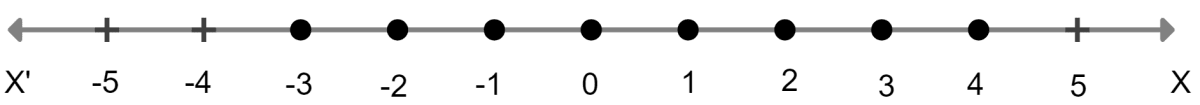#### Question 34

Find the greatest integer which is such that if 7 is added to its double, the resulting number becomes greater than three times the integer.

$\text{Let the greatest integer} = x \\[0.5em] \text{According to the condition}, \\[0.5em] 2x + 7 \gt 3x \\[0.5em] \Rightarrow 2x - 3x \gt - 7 \\[0.5em] \Rightarrow -x \gt - 7 \\[0.5em] \Rightarrow x \lt 7 \\[0.5em] \therefore \text{Value of x which is greatest = 6}$

#### Question 35

One-third of a bamboo pole is buried in mud, one-sixth of it is in water and the part above the water is greater than or equal to 3 metres. Find the length of the shortest pole.

Let the length of the shortest pole = x metre

Length of pole which is burried in mud = $\dfrac{x}{3}$

Length of pole which is in the water = $\dfrac{x}{6}$

Given,

$x-\Big[\dfrac{x}{3} + \dfrac{x}{6}\Big] \ge 3 \\[0.5em] \Rightarrow x - \Big(\dfrac{2x + x}{6}\Big) \ge 3 \\[0.5em] \Rightarrow x - \Big(\dfrac{3x}{6}\Big) \ge 3 \\[0.5em] \Rightarrow x - \dfrac{x}{2} \ge 3 \\[0.5em] \Rightarrow \dfrac{x}{2} \ge 3 \\[0.5em] \Rightarrow x \ge 6$

∴ Length of pole which is shortest = 6 meters.

## Multiple Choice Questions

#### Question 1

If x ∈ {-3, -1, 0, 1, 3, 5}, then the solution set of the inequation 3x – 2 ≤ 8 is

1. {-3, -1, 1, 3}
2. {-3, -1, 0, 1, 3}
3. {-3, -2, -1, 0, 1, 2, 3}
4. {-3, -2, -1, 0, 1, 2}

Given,

x ∈ {-3, -1, 0, 1, 3, 5}

$3x - 2 \le 8 \\[0.5em] \Rightarrow 3x \le 8 + 2 \\[0.5em] \Rightarrow 3x \le 10 \\[0.5em] \Rightarrow x \le \dfrac{10}{3} \\[0.5em] \Rightarrow x \le 3\dfrac{1}{3}$

Solution set = {-3, -1, 0, 1, 3}

∴ Option 2 is the correct option.

#### Question 2

If x ∈ W, then the solution set of the inequation 3x + 11 ≥ x + 8 is

1. {-2, -1, 0, 1, 2, …}
2. {-1, 0, 1, 2, …}
3. {0, 1, 2, 3, …}
4. {x : x ∈ R, x ≥ –$\dfrac{3}{2}$ }

x ∈ W

$3x + 11 \ge x + 8 \\[0.5em] \Rightarrow 3x - x \ge 8 – 11 \\[0.5em] \Rightarrow 2x \ge -3 \\[0.5em] \Rightarrow x \ge -\dfrac{3}{2} \\[0.5em]$

Solution set = {0, 1, 2, 3, …}

∴ Option 3 is the correct option.

#### Question 3

If x ∈ W, then the solution set of the inequation 5 - 4x ≤ 2 - 3x is

1. {…, -2, -1, 0, 1, 2, 3}
2. {1, 2, 3}
3. {0, 1, 2, 3}
4. {x : x ∈ R, x ≤ 3}

x ∈ W

$5 - 4x \le 2 - 3x \\[0.5em] \Rightarrow -4x + 3x \le 2 - 5 \\[0.5em] \Rightarrow -x \le -3 \\[0.5em] \Rightarrow x \ge 3$

Solution set = {3, 4, 5, ..... }.

∴ No option is correct .

#### Question 4

If x ∈ I, then the solution set of the inequation 1 < 3x + 5 ≤ 11 is

1. { -1, 0, 1, 2}
2. { -2, -1, 0, 1}
3. { -1, 0, 1}
4. {x : x ∈ R, -$\dfrac{4}{3}$ $\lt$ x $\le$ 2}

x ∈ I

Given,
1 < 3x + 5 ≤ 11

Solving left side,

$\Rightarrow 1 \lt 3x + 5 \\[0.5em] \Rightarrow 1 - 5 \lt 3x \\[0.5em] \Rightarrow -4 \lt 3x \\[0.5em] \Rightarrow 3x \gt -4 \\[0.5em] \Rightarrow x \gt -\dfrac{4}{3}$

Solving right side,

$3x + 5 \le 11 \\[0.5em] \Rightarrow 3x \le 11-5 \\[0.5em] \Rightarrow 3x \le 6 \\[0.5em] \Rightarrow x \le 2 \\[1.5em] \therefore -\dfrac{4}{3} \lt x \le 2$

Solution set = {-1, 0, 1, 2}.

∴ Option 1 is the correct option.

#### Question 5

If x ∈ R, the solution set of 6 ≤ -3(2x - 4) < 12 is

1. {x : x ∈ R, 0 < x ≤ 1}
2. {x : x ∈ R, 0 ≤ x < 1}
3. {0, 1}
4. none of these

x ∈ R

Given,
6 ≤ -3(2x - 4) < 12

Solving left side,

$6 \le -3(2x - 4) \\[0.5em] \Rightarrow 6 \le - 6x + 12 \\[0.5em] \Rightarrow 6-12 \le -6x \\[0.5em] \Rightarrow -6 \le -6x \\[0.5em] \Rightarrow 6x \le 6 \\[0.5em] \Rightarrow x \le 1$

Solving right side,

$-3(2x - 4) \lt 12 \\[0.5em] \Rightarrow -6x + 12 \lt 12 \\[0.5em] \Rightarrow -6x \lt 12-12 \\[0.5em] \Rightarrow -6x \lt 0 \\[0.5em] \Rightarrow x \gt 0 \\[1.5em] \therefore 0 \lt x \le 1$

Solution set = {x : x ∈ R, 0 $\lt x \le$ 1}

∴ Option 1 is the correct option.

## Chapter Test

#### Question 1

Solve the inequation : 5x - 2 ≤ 3(3 - x) where x ∈ { -2, -1, 0, 1, 2, 3, 4}. Also represent its solution on the number line.

Given,

$5x - 2 \le 3(3 - x) \\[0.5em] \Rightarrow 5x - 2 \le 9 - 3x \\[0.5em] \Rightarrow 5x + 3x \le 9 + 2 \\[0.5em] \Rightarrow 8x \le 11 \\[0.5em] \Rightarrow x \le \dfrac{11}{8}$

Since, x ∈ { -2, -1, 0, 1, 2, 3, 4}.

∴ Solution set ={-2, -1, 0, 1}.

The graph of the solution set is represented by thick black dots.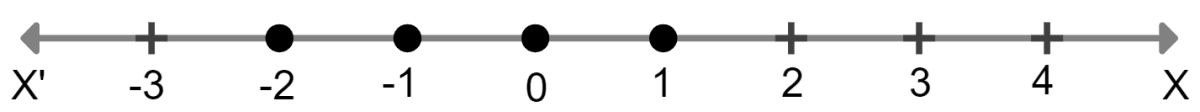#### Question 2

Solve the inequations : 6x - 5 < 3x + 4, x ∈ I

Given,

$6x - 5 \lt 3x + 4 \\[0.5em] \Rightarrow 6x - 3x \lt 4 + 5 \\[0.5em] \Rightarrow 3x \lt 9 \\[0.5em] \Rightarrow x \lt 3$

x ∈ I

∴ Solution Set = {..., -2, -1, 0, 1, 2}.

#### Question 3

Find the solution set of the inequation x + 5 ≤ 2x + 3 ; x ∈ R. Graph the solution set on the number line.

Given,

$x + 5 \le 2x + 3 \\[0.5em] \Rightarrow x - 2x \le 3 - 5\\[0.5em] \Rightarrow -x \le -2\\[0.5em] \Rightarrow x \ge 2$

∴ Solution set = {x : x ∈ R, x ≥ 2 }.

The graph of the solution set is represented by thick line starting from and including 2.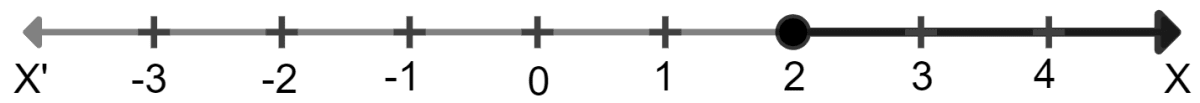#### Question 4

If x ∈ R (real numbers) and -1 < 3 - 2x ≤ 7, find solution set and represent it on a number line.

Given,

$-1 \lt 3 - 2x \le 7 \\[0.5em] \Rightarrow -1 \lt 3 - 2x \text{ and } 3 - 2x \le 7 \\[0.5em] \Rightarrow 2x \lt 3 + 1 \text{ and } -2x \le 7 - 3 \\[0.5em] \Rightarrow 2x \lt 4 \text{ and } -2x \le 4 \\[0.5em] \Rightarrow x \lt 2 \text{ and } -x \le 2 \\[0.5em] \Rightarrow x \lt 2 \text{ or } x \ge -2$

∴ Solution set = {x : x ∈ R, -2 ≤ x < 2}.

The graph of this inequation is represented by thick black line starting from -2 (including -2) till (not including) 2.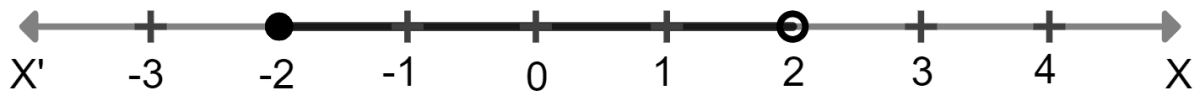#### Question 5

Solve the inequation :

$\dfrac{5x+1}{7} - 4\Big(\dfrac{x}{7}+ \dfrac{2}{5}\Big) \le 1\dfrac{3}{5}+\dfrac{3x-1}{7}, x ∈ \bold{R}.$

Given,

$\dfrac{5x+1}{7} - 4\Big(\dfrac{x}{7}+ \dfrac{2}{5}\Big) \le 1\dfrac{3}{5}+\dfrac{3x-1}{7}, x ∈ \bold{R}.$

Multiplying both sides by 35

$\Rightarrow 25x + 5-4(5x + 14) \le 56 + 15x -5 \\[0.5em] \Rightarrow 25x + 5 - 20x - 56 \le 56 + 15x -5 \\[0.5em] \Rightarrow 5x - 51 \le 51 + 15x \\[0.5em] \Rightarrow 5x - 15x \le 51 + 51 \\[0.5em] \Rightarrow -10x \le 102 \\[0.5em] \Rightarrow x \ge -\dfrac{102}{10} \\[0.5em] \Rightarrow x \ge -\dfrac{51}{5}$

∴ Solution set = {x : x ∈ R, x $\ge -\dfrac{51}{5}$ }.

#### Question 6

Find the range of values of x, which satisfy 7 ≤ –4x + 2 < 12, x ∈ R. Graph these values of x on the real number line.

Given,
7 ≤ –4x + 2 < 12

$\Rightarrow 7 \le - 4x + 2 \text{ and } - 4x + 2 \lt 12 \\[0.5em]$

Solving left side,

$7 \le - 4x + 2 \\[0.5em] \Rightarrow 4x \le 2-7 \\[0.5em] \Rightarrow 4x \le -5 \\[0.5em] \Rightarrow x \le \dfrac{-5}{4}$

Solving right side,

$-4x + 2 \lt 12 \\[0.5em] \Rightarrow -4x \lt 12 - 2 \\[0.5em] \Rightarrow -4x \lt 10 \\[0.5em] \Rightarrow 4x \gt -10 \\[0.5em] \Rightarrow x \gt \dfrac{-5}{2}$

∴ Solution set = {x : x ∈ R, $-\dfrac{5}{2}$ < x ≤ $-\dfrac{5}{4}$}.

The graph of the inequation is represented by thick black line starting from $-\dfrac{5}{2}$ (excluding $-\dfrac{5}{2}$) till $-\dfrac{5}{4}$ (including $-\dfrac{5}{4}$).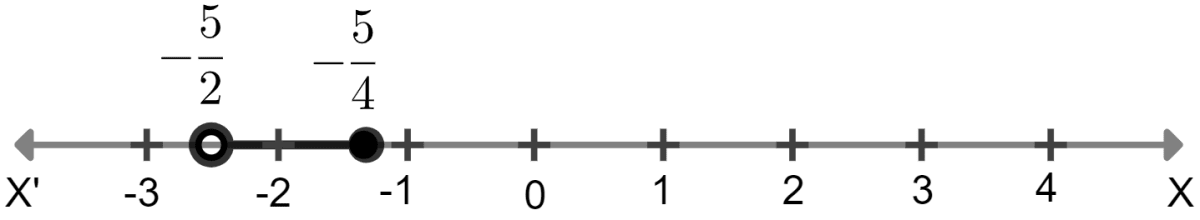#### Question 7

If x ∈ R, solve $2x - 3 \ge x+\dfrac{1−x}{3} \gt \dfrac{2}{5}x$. Also represent the solution on the number line.

Given,

$2x-3 \ge x + \dfrac{1-x}{3} \gt \dfrac{2}{5}x$

Solving left side,

$2x-3 \ge x + \dfrac{1-x}{3} \\[0.5em] \Rightarrow 2x - 3 \ge \dfrac{3x +1 -x}{3} \\[0.5em] \Rightarrow 2x -3 \ge \dfrac{2x + 1}{3} \\[0.5em] \Rightarrow 3 (2x -3) \ge 2x+1 \\[0.5em] \Rightarrow 6x - 9 \ge 2x + 1 \\[0.5em] \Rightarrow 6x - 2x \ge 1+ 9 \\[0.5em] \Rightarrow 4x \ge 10 \\[0.5em] \Rightarrow x \ge \dfrac{10}{4} \\[0.5em] \Rightarrow x \ge \dfrac{5}{2}$

Solving right side,

$x + \dfrac{1-x}{3} \gt \dfrac{2}{5}x \\[0.5em] \Rightarrow \dfrac{3x + 1 - x}{3} \gt \dfrac{2}{5}x \\[0.5em] \Rightarrow \dfrac{2x + 1}{3} \gt \dfrac{2}{5}x \\[0.5em] \Rightarrow 5(2x +1) \gt 6x \\[0.5em] \Rightarrow 10x + 5 \gt 6x \\[0.5em] \Rightarrow 10x - 6x \gt -5 \\[0.5em] \Rightarrow 4x \gt -5 \\[0.5em] \Rightarrow x \gt -\dfrac{5}{4}$

From left side we get $x \ge \dfrac{5}{2}$ and from right side we get $x \gt -\dfrac{5}{4}$

$x \ge \dfrac{5}{2}$

∴ Solution set = {x : x ∈ R, x $\ge \dfrac{5}{2}$ }

The graph of the inequation is represented by thick black line starting from $\dfrac{5}{2}$ (including $\dfrac{5}{2}$).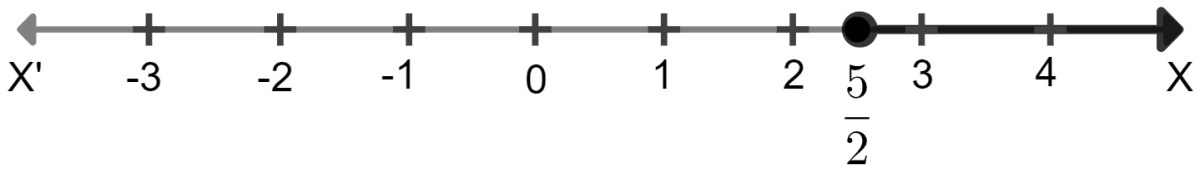#### Question 8

Find positive integers which are such that if 6 is subtracted from five times the integer then the resulting number cannot be greater than four times the integer.

Let the positive integer = x
According to the problem,

$5x - 6 \lt 4x \\[0.5em] \Rightarrow 5x - 4x \lt 6 \\[0.5em] \Rightarrow x \lt 6$

∴ Solution set = { 1, 2, 3, 4, 5, 6}.

#### Question 9

Find three smallest consecutive natural numbers such that the difference between one-third of the largest and one-fifth of the smallest is at least 3.

Let first least natural number = x
then, second number = x + 1
and third number = x + 2

Given,

$\dfrac{1}{3}(x+2) - \dfrac{1}{5}(x) \ge 3 \\[0.5em] \Rightarrow \dfrac{x}{3} - \dfrac{x}{5} \ge 3 - \dfrac{2}{3} \\[0.5em] \Rightarrow \dfrac{5x- 3x}{15} \ge \dfrac{9-2}{3} \\[0.5em] \Rightarrow \dfrac{2x}{15} \ge \dfrac{7}{3} \\[0.5em] \Rightarrow 2x \ge \dfrac{7 \times 15}{3} \\[0.5em] \Rightarrow 2x \ge 35 \\[0.5em] \Rightarrow x \ge \dfrac{35}{2} \\[0.5em] \Rightarrow x \ge 17\dfrac{1}{2}$

Since the three consecutive numbers should be natural numbers
∴ x = 18
x + 1 = 19
x + 2 = 20

Hence, the three smallest consecutive natural numbers are 18, 19, 20